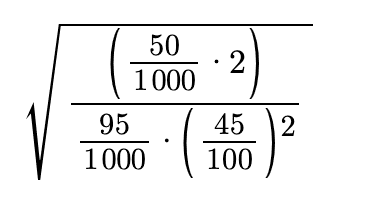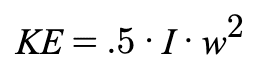# Angular Velocity from KE, radius, and mass

aivilo775
Homework Statement:
A 45-cm-long, 95 g rod rotates about an axle at one end of the rod. At what angular velocity, in rpm , does the rod have 50 mJ of rotational kinetic energy?
Relevant Equations:
Not given
I tried using the equation w^2 = (4*K)/(mr^2) but I don't think this is right... I got my answer to be 3.2243 and that's not correct

Staff Emeritus
Homework Helper
Gold Member
Homework Statement::
A 45-cm-long, 95 g rod rotates about an axle at one end of the rod. At what angular velocity, in rpm , does the rod have 50 mJ of rotational kinetic energy?
Relevant Equations:: Not given

I tried using the equation w^2 = (4*K)/(mr^2) but I don't think this is right... I got my answer to be 3.2243 and that's not correct
Hello @aivilo775 ,Under Relevant Equations, you should supply whatever equations are relevant to your problem, such as: ##KE=(1/2)I\omega^2##
for the problem you posted.

Also of importance is what you use to get the moment of inertia, ##I## .

You need to be more detailed as to how you obtained your result. Also be careful with units and define symbols.

•PhDeezNutz and topsquark
aivilo775This is the math I did when I rearrangedto solve for w, angular velocity. I used I = mr^2. I got w = 2.27995. The units would be the sqrt of(J /kg*m^2), which ends up just being 1/sec. to get the answer in rpm, I figured I would multiply w by 60sec (to get w = 136.797, but this wasn't right

Homework Helper
Gold Member
Please use LaTeX to write your equations in symbolic form. It is not at all clear what the numbers you posted in #3 are all about. Also, what is the moment of inertia of the rod? Look it up.

•topsquark
•